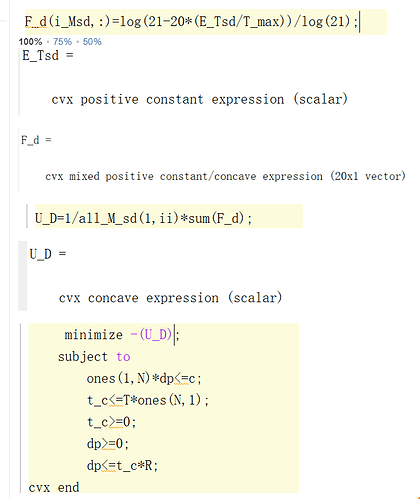# Failed Status and optimal value NAN？？

##image.png693×824 29.9 KB 18/ 19 | 1.041e-01 1.159e+01s 0.000e+00 | Solved

Status: Failed
Optimal value (cvx_optval): NaN

Successive Approximation method is still invoked.Can you help me see where is the problem?

You haven’t shown your entire program, so I am not sure what is what. But it appears that you still have instances of `exp(cvx_expression)`, which you should deal with as shown iny link above.

In the future, rather than posting an image of your code, please copy and paste it into your post, and use the Pre-formatted text icon. That allows forum readers to copy and paste the code into their own MATLAB session.

``````for ii=1:length(all_M_sd)
cvx_begin
clear F_d F_dp F_q q_sd ;
*variables t_c(N) dp(N) z1 z2;*
rsuid_sdall(ii,:)=sum(rsu_sd(1:all_M_sd(1,ii),:));
for i_Msd=1:all_M_sd(1,ii)
d_sd=Z_M{1,i_Msd}(:,42);
u_sd=Z_M{1,i_Msd}(:,41);
S_sd=Z_M{1,i_Msd}(:,1:40);
X_sd=zeros(N,1);
X_sd((1:(N-n_sd(i_Msd,1))),:)=1;
E_Tsd=0;
for i=1:N
*{(S_sd(i,:)*t_c*u_sd(i,1)-X_sd(i,1)*(T*(1+exp(T))+exp(-T))),1,z1} == exponential(1);*
*E_Tsd0=T+(d_sd(i,1)-2*r)/v+(T*exp(-u_sd(i,1)*T)+exp(-u_sd(i,1)*T)/u_sd(i,1))*z1;*
*E_Tsd=E_Tsd+E_Tsd0;*
end
T_max=2*ones(1,N)*(T*ones(N,1)+(d_sd-ones(N,1)*2*r)/v);
*F_d(i_Msd,:)=(21-20*(E_Tsd/T_max));*
*dp_sd=sum(S_sd*dp);*
*{(5*((sum(S_sd*dp))/(n_sd(i_Msd,1))/T/R)-1),1,z2} == exponential(1);*
*F_dp(i_Msd,:)=1-z2;*
end
*U_D=sum(-rel_entr(1,F_d));*
*U_dp=1/all_M_sd(1,ii)*sum(F_dp);*
minimize -(U_D+U_dp);
subject to
ones(1,N)*dp<=c;
t_c<=T*ones(N,1);
t_c>=0;
dp>=0;
dp<=t_c*R;
cvx_end
U(ii,:)=[U_D U_dp ];
Utility(ii,1)=(U_D+U_dp);
dp_all(:,ii)=dp;
t_c_all(:,ii)=t_c;
end`Preformatted text`
``````

The objective function is somewhat complicated, involving exp and log, variables t_c, dp, and the rest are known matrices or numbers. E_Tsd is an exp expression on t_c that is summed by loops. U_D needs to sum the F_d after the log. F_dp is a log of the expression of the dp variable. Only the exp or log expression containing the variable is processed, and the Using Pade approximation for exponential cone with parameters m=3, k=3 appears in the program, but the final solution is still invoked with the Successive Approximation method.

I suggest for every instance of `log` and `exp` in your CVX program, just prior to the statement in which it appears, examine the argument of `log` and `exp` by typing the argument at the command line. Look for any of the arguments which are not constants (numerical). I.e., any argument which is a CVX expression of any kind. Those need to be replaced per the previously provided link in order to avoid use of the Successive Approximation Method.

# Using Pade approximation for exponential cone with parameters m=3, k=3

## Calling SDPT3 4.0: 31377 variables, 9929 equality constraints For improved efficiency, SDPT3 is solving the dual problem.

num. of constraints = 9929
dim. of sdp var = 19692, num. of sdp blk = 9846
dim. of linear var = 1802
dim. of free var = 37 *** convert ublk to lblk

SDPT3: Infeasible path-following algorithms

## number of iterations = 5 Total CPU time (secs) = 3.30 CPU time per iteration = 0.66 termination code = 3 DIMACS: 8.0e+07 0.0e+00 6.8e+00 0.0e+00 1.0e+00 9.6e-50

Status: Failed
Optimal value (cvx_optval): NaN
Still failing, what could be the problem?

This shows that you have succeeded in having the Successive Approximation method not be used.

Perhaps you have a numerically difficult (bad) problem. The first thing to check is the scaling of the data (input data should be within a small number of orders of magnitude of 1). It is also possible you made a mistake.

I recommend you try using SeDuMi as the solver and see how that works. The best solvers to use, if they are available to you, are Mosek and Gurobi, which are likely better able to handle numerically difficult problems than SDPT3 or SeDuMi.

One other thing you could try, but I don;t think will necessarily help is to increase the values of m and k used by CVXQUAD ( I think that might require modifying the source code of CVXQUAD).

``````Calling SeDuMi 1.34: 31379 variables, 9929 equality constraints
For improved efficiency, SeDuMi is solving the dual problem.
------------------------------------------------------------
SeDuMi 1.34 (beta) by AdvOL, 2005-2008 and Jos F. Sturm, 1998-2003.
Alg = 2: xz-corrector, Adaptive Step-Differentiation, theta = 0.250, beta = 0.500
Split 39 free variables
eqs m = 9929, order n = 21573, dim = 31419, blocks = 9847
nnz(A) = 43714 + 0, nnz(ADA) = 44029, nnz(L) = 30373
it :     b*y       gap    delta  rate   t/tP*  t/tD*   feas cg cg  prec
0 :            2.35E+51 0.000
1 :  -2.06E+55 5.83E+50 0.000 0.2476 0.9000 0.9000  -0.63 33  8  4.3E+03
2 :  -1.29E+56 3.42E-54 0.000 0.0000 0.8649 0.9000  -0.89 99 99  4.2E+03
3 :  -7.10E+56 7.24E-55 0.000 0.2118 0.9000 0.9000  -0.98 99 99  4.2E+03
4 :  -2.88E+57 1.91E-55 0.000 0.2636 0.9000 0.9000  -0.99 99 99  4.1E+03
5 :  -1.06E+58 4.82E-56 0.000 0.2527 0.9015 0.9000  -1.00 99 99  4.0E+03
6 :  -3.78E+58 1.40E-56 0.000 0.2896 0.9007 0.9000  -1.01 99 99  4.2E+03
7 :  -4.05E+58 4.13E-57 0.000 0.2960 0.9010 0.9000  -1.10 99 99  4.4E+03
Run into numerical problems.

Dual infeasible, primal improving direction found.
iter seconds  |Ax|    [Ay]_+     |x|       |y|
7     15.1   2.1e-56   3.3e+50   2.5e-53   8.2e+54

Detailed timing (sec)
Pre          IPM          Post
2.110E-01    5.225E+00    2.099E-02
Max-norms: ||b||=1, ||c|| = 7.393585e+54,
Cholesky |add|=0, |skip| = 9929, ||L.L|| = 1.
------------------------------------------------------------
Status: Infeasible
Optimal value (cvx_optval): +Inf``````
``````Calling Mosek 8.0.0.60: 15779 variables, 5009 equality constraints
For improved efficiency, Mosek is solving the dual problem.
------------------------------------------------------------

MOSEK Version 8.0.0.60 (Build date: 2017-3-1 13:09:33)
Copyright (c) MOSEK ApS, Denmark. WWW: mosek.com
Platform: Windows/64-X86

Mosek error: MSK_RES_ERR_HUGE_C (A large value of 1.5e+054 has been specified in cx for variable '' (0).)
------------------------------------------------------------
Status: Error
Optimal value (cvx_optval): NaN
``````

[ x, status, tprec, iters, y ] = shim.solve( At, b, c, cones, quiet, prec, solv.settings, eargs{:}
);

solve( prob );

cvx_end

You need to rescale the problem. No solver can handle coefficients of order 1.5e+054 and MOSEK explicitly tells you it will not cooperate. If you are working with exponential functions then maybe you accidentally formulated something like e^{100}.

1 Like